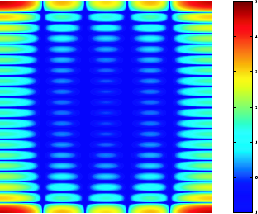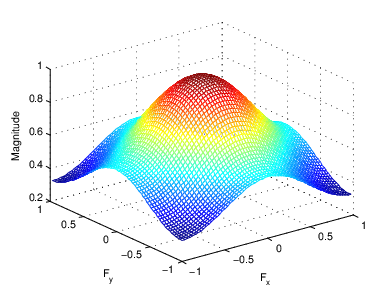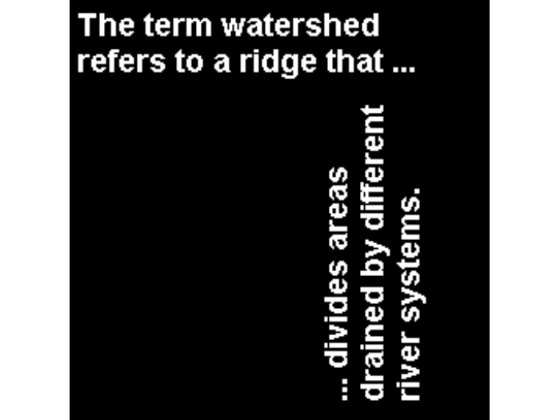Fourier Transform

Definition of Fourier Transform

The Fourier transform is a representation of an image as a sum of complex exponentials of varying magnitudes, frequencies, and phases. The Fourier transform plays a critical role in a broad range of image processing applications, including enhancement, analysis, restoration, and compression.

If f(m,n) is a function of two discrete spatial variables m and n, then the two-dimensional Fourier transform of f(m,n) is defined by the relationship

$F\left({\omega }_{1},{\omega }_{2}\right)=\sum _{m=-\infty }^{\infty }\sum _{n=-\infty }^{\infty }f\left(m,n\right){e}^{-j{\omega }_{1}m}{e}^{-j{\omega }_{2}n}.$

The variables ω1 and ω2 are frequency variables; their units are radians per sample. F(ω1,ω2) is often called the frequency-domain representation of f(m,n). F(ω1,ω2) is a complex-valued function that is periodic both in ω1 and ω2, with period $2\pi$. Because of the periodicity, usually only the range $-\pi \le {\omega }_{1},{\omega }_{2}\le \pi$ is displayed. Note that F(0,0) is the sum of all the values of f(m,n). For this reason, F(0,0) is often called the constant component or DC component of the Fourier transform. (DC stands for direct current; it is an electrical engineering term that refers to a constant-voltage power source, as opposed to a power source whose voltage varies sinusoidally.)

The inverse of a transform is an operation that when performed on a transformed image produces the original image. The inverse two-dimensional Fourier transform is given by

$f\left(m,n\right)=\frac{1}{4{\pi }^{2}}{\int }_{{\omega }_{1}=-\pi }^{\pi }{\int }_{{\omega }_{2}=-\pi }^{\pi }F\left({\omega }_{1},{\omega }_{2}\right){e}^{j{\omega }_{1}m}{e}^{j{\omega }_{2}n}d{\omega }_{1}d{\omega }_{2}.$

Roughly speaking, this equation means that f(m,n) can be represented as a sum of an infinite number of complex exponentials (sinusoids) with different frequencies. The magnitude and phase of the contribution at the frequencies (ω1,ω2) are given by F(ω1,ω2).

Visualizing the Fourier Transform

To illustrate, consider a function f(m,n) that equals 1 within a rectangular region and 0 everywhere else. To simplify the diagram, f(m,n) is shown as a continuous function, even though the variables m and n are discrete.

Rectangular FunctionThe following figure shows, as a mesh plot, the magnitude of the Fourier transform,

$|F\left({\omega }_{1},{\omega }_{2}\right)|,$

of the rectangular function shown in the preceding figure. The mesh plot of the magnitude is a common way to visualize the Fourier transform.

Magnitude Image of a Rectangular FunctionThe peak at the center of the plot is F(0,0), which is the sum of all the values in f(m,n). The plot also shows that F(ω1,ω2) has more energy at high horizontal frequencies than at high vertical frequencies. This reflects the fact that horizontal cross sections of f(m,n) are narrow pulses, while vertical cross sections are broad pulses. Narrow pulses have more high-frequency content than broad pulses.

Another common way to visualize the Fourier transform is to display

$\mathrm{log}|F\left({\omega }_{1},{\omega }_{2}\right)|$

as an image, as shown.

Log of the Fourier Transform of a Rectangular FunctionUsing the logarithm helps to bring out details of the Fourier transform in regions where F(ω1,ω2) is very close to 0.

Examples of the Fourier transform for other simple shapes are shown below.

Fourier Transforms of Some Simple ShapesDiscrete Fourier Transform

Working with the Fourier transform on a computer usually involves a form of the transform known as the discrete Fourier transform (DFT). A discrete transform is a transform whose input and output values are discrete samples, making it convenient for computer manipulation. There are two principal reasons for using this form of the transform:

• The input and output of the DFT are both discrete, which makes it convenient for computer manipulations.

• There is a fast algorithm for computing the DFT known as the fast Fourier transform (FFT).

The DFT is usually defined for a discrete function f(m,n) that is nonzero only over the finite region $0\le m\le M-1$ and $0\le n\le N-1$. The two-dimensional M-by-N DFT and inverse M-by-N DFT relationships are given by

and

The values F(p,q) are the DFT coefficients of f(m,n). The zero-frequency coefficient, F(0,0), is often called the "DC component." DC is an electrical engineering term that stands for direct current. (Note that matrix indices in MATLAB® always start at 1 rather than 0; therefore, the matrix elements f(1,1) and F(1,1) correspond to the mathematical quantities f(0,0) and F(0,0), respectively.)

The MATLAB functions fft, fft2, and fftn implement the fast Fourier transform algorithm for computing the one-dimensional DFT, two-dimensional DFT, and N-dimensional DFT, respectively. The functions ifft, ifft2, and ifftn compute the inverse DFT.

Relationship to the Fourier Transform

The DFT coefficients F(p,q) are samples of the Fourier transform F(ω1,ω2).

$\begin{array}{cc}F\left(p,q\right)=F\left({\omega }_{1},{\omega }_{2}\right){|}_{\begin{array}{l}{\omega }_{1}=2\pi p/M\\ {\omega }_{2}=2\pi q/N\end{array}}& \begin{array}{l}p=0,1,...,M-1\\ q=0,1,...,N-1\end{array}\end{array}$

Visualizing the Discrete Fourier Transform

1. Construct a matrix f that is similar to the function f(m,n) in the example in Definition of Fourier Transform. Remember that f(m,n) is equal to 1 within the rectangular region and 0 elsewhere. Use a binary image to represent f(m,n).

f = zeros(30,30);
f(5:24,13:17) = 1;
imshow(f,'InitialMagnification','fit')2. Compute and visualize the 30-by-30 DFT of f with these commands.

F = fft2(f);
F2 = log(abs(F));
imshow(F2,[-1 5],'InitialMagnification','fit');
colormap(jet); colorbar

Discrete Fourier Transform Computed Without PaddingThis plot differs from the Fourier transform displayed in Visualizing the Fourier Transform. First, the sampling of the Fourier transform is much coarser. Second, the zero-frequency coefficient is displayed in the upper left corner instead of the traditional location in the center.

3. To obtain a finer sampling of the Fourier transform, add zero padding to f when computing its DFT. The zero padding and DFT computation can be performed in a single step with this command.

F = fft2(f,256,256);

This command zero-pads f to be 256-by-256 before computing the DFT.

imshow(log(abs(F)),[-1 5]); colormap(jet); colorbar

Discrete Fourier Transform Computed with Padding4. The zero-frequency coefficient, however, is still displayed in the upper left corner rather than the center. You can fix this problem by using the function fftshift, which swaps the quadrants of F so that the zero-frequency coefficient is in the center.

F = fft2(f,256,256);F2 = fftshift(F);
imshow(log(abs(F2)),[-1 5]); colormap(jet); colorbar

The resulting plot is identical to the one shown in Visualizing the Fourier Transform.

Applications of the Fourier Transform

This section presents a few of the many image processing-related applications of the Fourier transform.

Frequency Response of Linear Filters

The Fourier transform of the impulse response of a linear filter gives the frequency response of the filter. The function freqz2 computes and displays a filter's frequency response. The frequency response of the Gaussian convolution kernel shows that this filter passes low frequencies and attenuates high frequencies.

h = fspecial('gaussian');
freqz2(h)

Frequency Response of a Gaussian FilterSee Design Linear Filters in the Frequency Domain for more information about linear filtering, filter design, and frequency responses.

Perform Fast Convolution Using the Fourier Transform

This example shows how to perform fast convolution of two matrices using the Fourier transform. A key property of the Fourier transform is that the multiplication of two Fourier transforms corresponds to the convolution of the associated spatial functions. This property, together with the fast Fourier transform, forms the basis for a fast convolution algorithm.

Note: The FFT-based convolution method is most often used for large inputs. For small inputs it is generally faster to use the imfilter function.

Create two simple matrices, A and B. A is an M-by-N matrix and B is a P-by-Q matrix.

A = magic(3);
B = ones(3);

Zero-pad A and B so that they are at least (M+P-1)-by-(N+Q-1). (Often A and B are zero-padded to a size that is a power of 2 because fft2 is fastest for these sizes.) The example pads the matrices to be 8-by-8.

A(8,8) = 0;
B(8,8) = 0;

Compute the two-dimensional DFT of A and B using the fft2 function. Multiply the two DFTs together and compute the inverse two-dimensional DFT of the result using the ifft2 function.

C = ifft2(fft2(A).*fft2(B));

Extract the nonzero portion of the result and remove the imaginary part caused by roundoff error.

C = C(1:5,1:5);
C = real(C)
C = 5×5

8.0000    9.0000   15.0000    7.0000    6.0000
11.0000   17.0000   30.0000   19.0000   13.0000
15.0000   30.0000   45.0000   30.0000   15.0000
7.0000   21.0000   30.0000   23.0000    9.0000
4.0000   13.0000   15.0000   11.0000    2.0000

Perform FFT-Based Correlation to Locate Image Features

This example shows how to use the Fourier transform to perform correlation, which is closely related to convolution. Correlation can be used to locate features within an image. In this context, correlation is often called template matching.

Read a sample image into the workspace.

Create a template for matching by extracting the letter "a" from the image. Note that you can also create the template by using the interactive syntax of the imcrop function.

a = bw(32:45,88:98);

Compute the correlation of the template image with the original image by rotating the template image by 180 degrees and then using the FFT-based convolution technique. (Convolution is equivalent to correlation if you rotate the convolution kernel by 180 degrees.) To match the template to the image, use the fft2 and ifft2 functions. In the resulting image, bright peaks correspond to occurrences of the letter.

C = real(ifft2(fft2(bw) .* fft2(rot90(a,2),256,256)));
figure
imshow(C,[]) % Scale image to appropriate display range.To view the locations of the template in the image, find the maximum pixel value and then define a threshold value that is less than this maximum. The thresholded image shows the locations of these peaks as white spots in the thresholded correlation image. (To make the locations easier to see in this figure, the example dilates the thresholded image to enlarge the size of the points.)

max(C(:))
ans = 68
thresh = 60; % Use a threshold that's a little less than max.
D = C > thresh;
se = strel('disk',5);
E = imdilate(D,se);
figure
imshow(E) % Display pixels with values over the threshold.